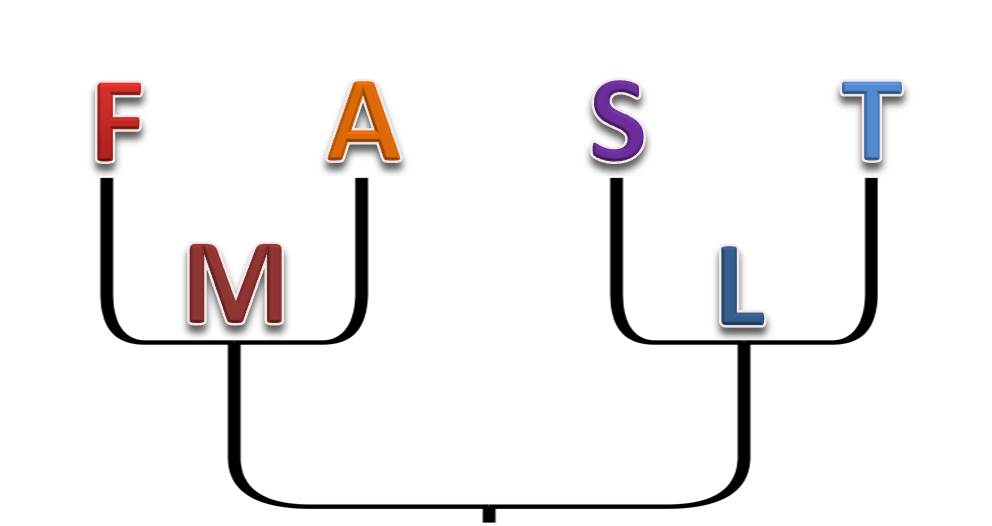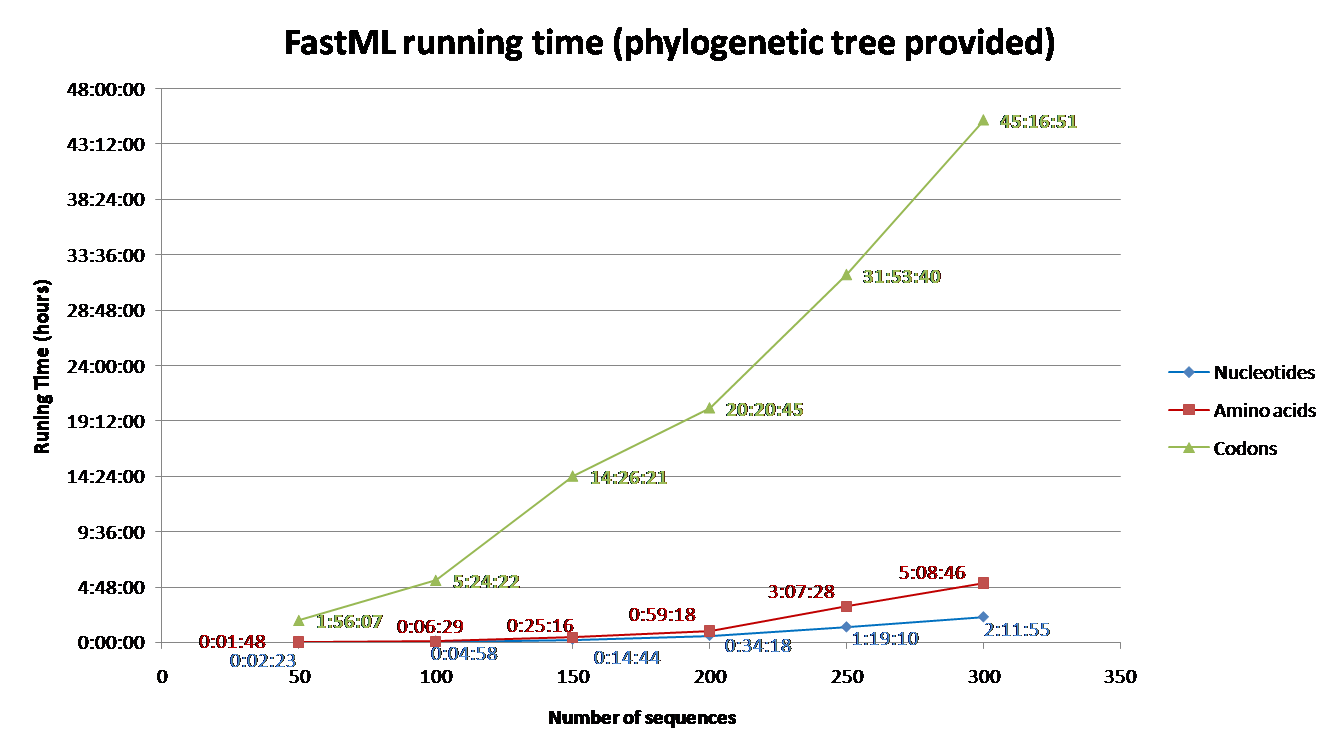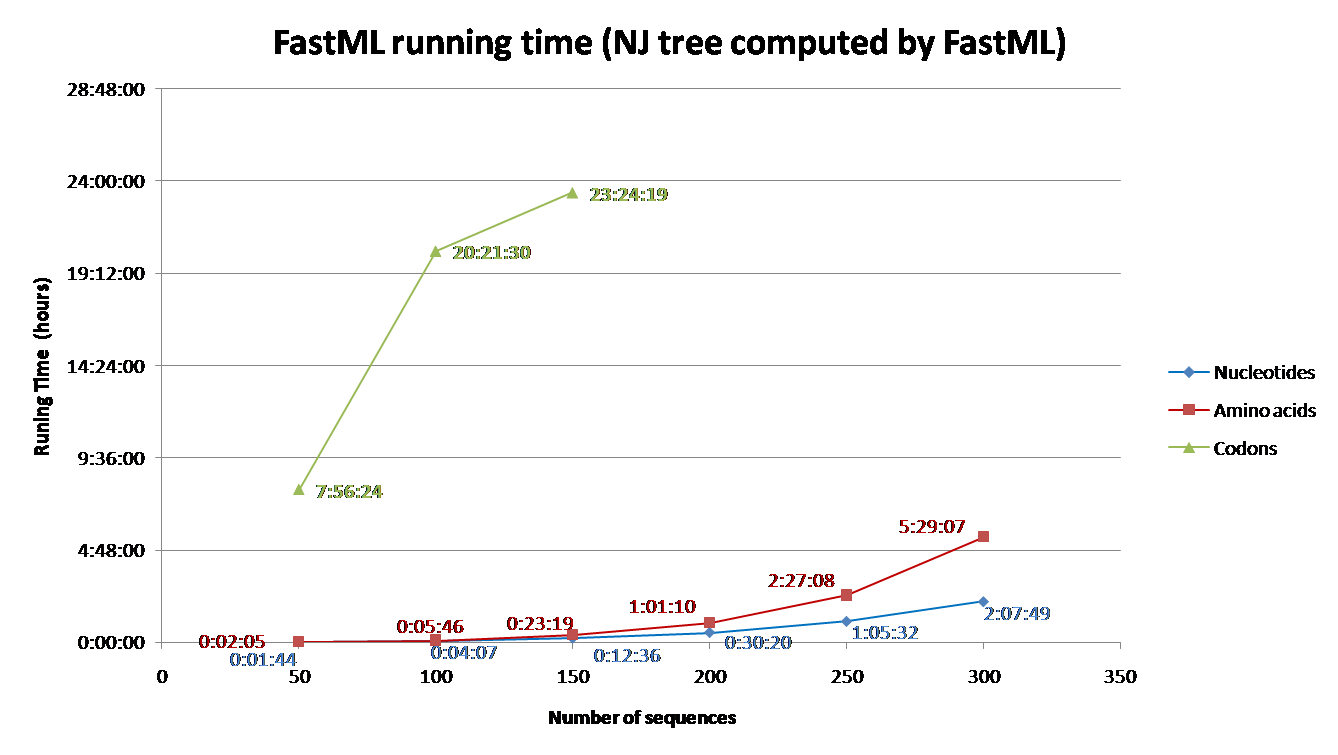The FASTML ServerServer for computing Maximum Likelihoodancestral sequence reconstruction HOME    OVERVIEW    GALLERY    SOURCE CODE    NEW FASTML PROGRAM    CITING & CREDITS    OLD VERSION

The FASTML Server - Server for computing Maximum Likelihood ancestral sequence reconstruction - overview

# FastML Overview

Introduction

The FastML server is a bioinformatics tool for the reconstruction of ancestral sequences based on the phylogenetic relations between homologous sequences. The server runs several algorithms that reconstruct the ancestral sequences with emphasis on an accurate reconstruction of both indels and characters. For character reconstruction the previously described FastML algorithms [1, 2] are used to efficiently infer the most likely ancestral sequences for each internal node of the tree. Both joint and the marginal reconstructions are provided. For indels reconstruction the sequences are first coded according to the indel events detected within the multiple sequence alignment (MSA)  and then a state-of-the-art likelihood model is used to reconstruct ancestral indels states [4, 5]. The server results are the most probable sequences, together with posterior probabilities for each character and indel at each sequence position for each internal node of the tree. FastML is generic and is applicable for any type of molecular sequences (nucleotide, protein, or codon sequences).

Methodology

Given a multiple sequence alignment (MSA) and optionally a phylogenetic tree, the ancestral reconstruction process can be divided into two parts:

1) Character reconstruction - two methods are implemented: the joint and the marginal. In the joint reconstruction, one finds the set of all the internal nodes sequences. In the marginal reconstruction, one infers the most likely sequence in a specific internal node. The results of these two estimation methods are not necessarily the same [1, 2]. Both methods are based on maximum likelihood (ML) algorithms and on an empirical Bayesian approach taking into account the rate variation among sites of the MSA.

2) Reconstruction of indels - a two steps approach is used in order to take into account the dependency among sites:

a. Indels coding.
In this step, the input MSA is coded into a binary indels matix. The server uses an efficient implementation of the simple indel coding  according to which each indel with different start and/or end positions is considered to be a separate character. All indels in the data are coded as binary (presence\absence) characters, each of which may represent a gap of multiple sites.

b. Indels reconstruction
In this step, the evolutionary analysis of indels is performed. Given the presence and absence binary matrix of indels in the extant sequences, the algorithms reconstructs the ancestral state of each indel in each internal node of the tree. It is assumed that the observed pattern of indels is the result of deletions and insertions dynamics along a phylogenetic tree. Our state-of-the-art inference methodology is based on a likelihood-based mixture model that allows variable rates of insertions and deletions among indel sites to reliably capture the underlying evolutionary processes [4, 5]. In this approach the posterior probability of indel presence (gap) is computed for each indel site and each node. Alternatively, users can select to reconstruct the ancestral states of the indels based on the maximum parsimony approach. The parsimonious ancestral reconstruction is based on the Sankoff algorithm . In this approach the parsimonious assignment of indel presence (gap) is computed for each indel and each internal node.

Running Time

Running time depends on the number of sequence and their length, the evolutionary model, the proportion of gaps, and the reconstruction algorithm. Codon models are the most time consuming and nucleotide models are the least. Additionally, accounting for among site rate variation is significantly more complex for the joint reconstruction, comparing to the marginal reconstruction . Additional info regarding the effect of each parameter on the running time is detailed below (under 'Advanced Options and Details' section). To aid users with estimating running time for their datasets, we simulated sequences using INDELible and computed their ancestral sequences using FastML.

The parameters used for the simulations were: codon model (M0 with kappa=2.5, omega=0.5); Power law insertion length distribution (a=3, M=60). Power law deletion length distribution (a=3.1, M=500); insertion rate = deletion rate = 0.01; The sequence length (without gaps) was set to be approximately 350; A random tree was generated for each simulation with parameters equal to: birth=1.1, death=0.2566 and sample mut=0.34. Varied numbers of sequences were simulated (See Fig. 1 and 2) based on the abovementioned parameters.

FastML was input with the simulated multiple sequence alignment and using (i) the simulated tree (Fig 1) or (ii) building NJ tree (Fig2). The running time for each of these scenarios was measured for (a) Amino Acid sequences (JTT model); (b) Nucleotides sequences (JC model) and (c) Codons (yang model). The average running time of FastML over twenty replicates of such simulated sequence are shown below. In addition, an estimation of the running time is given for each run.

FIGURE 1FIGURE 2

Input
FastML requires only an MSA as input. The MSA can be in any type of molecular sequences (nucleotide, protein, or codon sequences). The sequences may be in Fasta format only. If you are working with other sequence file formats such as Clustal, Phylip, etc., we suggest using software such as Bioedit to convert your format to Fasta.

• Generating the phylogenetic tree

The ancestral sequence reconstruction is based on a phylogeny that should be consistent with the input MSA. The user may provide a phylogenetic tree file (in Newick format) as input. Alternatively, the user is allowed to choose between two methods for phylogeny reconstruction: (1) The neighbor joining (NJ) algorithm  as implemented in the FastML program; or (2) a maximum likelihood (ML) algorithm as implemented in the RAxML program . In either case, the branch lengths are optimized based on the ML approach and using an expectation maximization (EM) algorithm [9, 10].

• Evolutionary models

FastML implements an assembly of different evolutionary models according the type of the molecular sequences.

For nucleotides, four evolutionary models are implemented in the FastML server: (i) the Juke and Cantor 69 model (JC69), which assumes equal base frequencies and equal substitution rates . (ii) The Tamura 92 model that uses only one parameter, which captures variation in G-C content . (iii) The HKY85 model, which distinguishes between transitions and transversions and allows unequal base frequencies . (iv) The General Time Reversible (GTR) model, which is the most general time-reversible model. The GTR parameters consist of an equilibrium base frequency vector, giving the frequency at which each base occurs at each site, and the rate matrix 

For amino acid sequences different matrices are available including: JTT , Dayhoff , WAG , and LG . The WAG matrix has been inferred from a large database of sequences comprising a broad range of protein families and is thus suited for distantly related amino acid sequences . The LG  matrix incorporates variability of evolutionary rates across sites and was shown to outperform other substitutions matrices for proteins. Moreover, two context dependents matrices are available: the mtREV  and cpREV  matrices that are suitable for mitochondrial, and chloroplast DNA-encoded proteins, respectively.

For codon sequences both the theoretical M5 model , the empirical codon matrix  and the MEC model  are implemented.

• Branch lengths optimization

In order to reduce the running time of the reconstruction, the user may turn off the optimization of branch lengths. Note that the accuracy of the branch lengths has a critical effect on the accuracy of the reconstruction. Therefore, this option is recommended for use only if the branch lengths are already optimized according to the same evolutionary model that is used for the reconstruction.

• Marginal reconstruction vs. joint reconstruction

The most likely reconstruction of the marginal and the joint maximum-likelihood methods may differ in some cases [see 1]. In marginal reconstruction, the most likely sequence at a specific internal node is inferred, averaging over all possible ancestral states at all other nodes. However, in joint reconstruction, the most likely set of ancestral states at all the internal nodes is inferred. When accounting for among site rate variation (using a gamma distribution, see below), the joint reconstruction time-complexity is exponential. Although in most practical scenarios both types of reconstruction are applicable for large datasets, turning off the joint reconstruction may speed up the algorithm.

• Accounting for among site rate variation

By default FastML uses a gamma distribution to model among site rate variation. The user may choose instead to assume an homogenous model, resulting in a much faster, yet less accurate, reconstruction.

Output

FastML directs you to a web page called "FastML Job Status Page". This web page is automatically updated every 30 seconds, showing messages regarding the different stages of the server activity. When the calculation finishes, several links appear.

1. Graphical representation of the entire reconstructed ancestral sequences: Using the Jalview multiple alignment editor  the server generates two windows: the first contains the MSA that was given as input to which the most probable ancestral sequences are added; the second contains the graphical representation of the phylogeny. Using these two windows, the server provides:

• A projection of the ancestral sequences onto the phylogeny: Internal nodes of the phylogenetic trees are numbered so that their associated reconstructed ancestral sequences can be easily located in the MSA.

• A colored-scaled projection of the reconstruction's confident: On the MSA window, the reconstructed ancestral sequences at each node are color-coded according to the posterior probabilities. Green represents a high confident while pink represents a relatively low one. In addition, by moving the cursor over the ancestral sequences, one can view the exact posterior probability of each node and each site.

• An option to download specific sequences of interest: Clicking on a specific node of interest on the phylogeny leads to the selection of all its descendent leaves, both on the tree and also in the MSA. The user can then download the sequences of these leaves by right-clicking any of the selected sequence's names on the MSA window and then clicking on "Selection" and "Output to Textbox". Similarly, the user can download the ancestral sequence reconstruction of a specific internal node by selecting it in the MSA window.

These graphical outputs are available for: (i) the characters joint reconstruction; (ii) the characters marginal reconstruction; and (iii) the integration of the marginal reconstruction together with the indel reconstruction.

2. Output files
1. Marginal reconstruction:

• Ancestral sequences according to the marginal reconstruction (including \ without reconstruction of indels): An MSA file (Fasta format) that contains the most probable ancestral sequences according to the marginal reconstruction.

• A graphical logo of the inferred ancestral sequences: Here we provide an option to generate for each ancestral node a logo figure using WebLogo  that represents the posterior probabilities of each reconstructed character, thus showing all possible alternative reconstructions in one figure.

• A set of the most likely ancestral sequences: Here we provide an option to generate for each ancestral node a set of the k most probable ancestral sequences for each ancestral node (where k is defined by the user).

• A sample of ancestral sequences: Here we provide an option to generate for each ancestral node a set of sequences sampled according to the posterior probabilities for each site (where l is defined by the user).

• Posterior probabilities of the most probable ancestral sequence reconstructions: The integrated posterior probability of each character and indel at each internal node.

• Posterior probability of each character at each site and each internal node. The possible reconstructed characters are ordered according to their probability, from the most likely to the least probable one.

• Posterior probability of each indel event at each ancestral node.

2. Joint reconstruction:
• Ancestral sequences according to the joint reconstruction: An MSA file (Fasta format) that contains the most probable ancestral sequences according to the joint reconstruction.

• Log likelihood values of the ancestral sequence reconstruction at each position.

3. Phylogeny outputs:
• Tree in Newick format: The reconstructed phylogeny in newick format, including the names used for the leaves and internal nodes.

• Tree in Ancestral format:The reconstructed phylogeny in ancestral format in which the parent node and the children nodes of each node of the tree are specified.

References